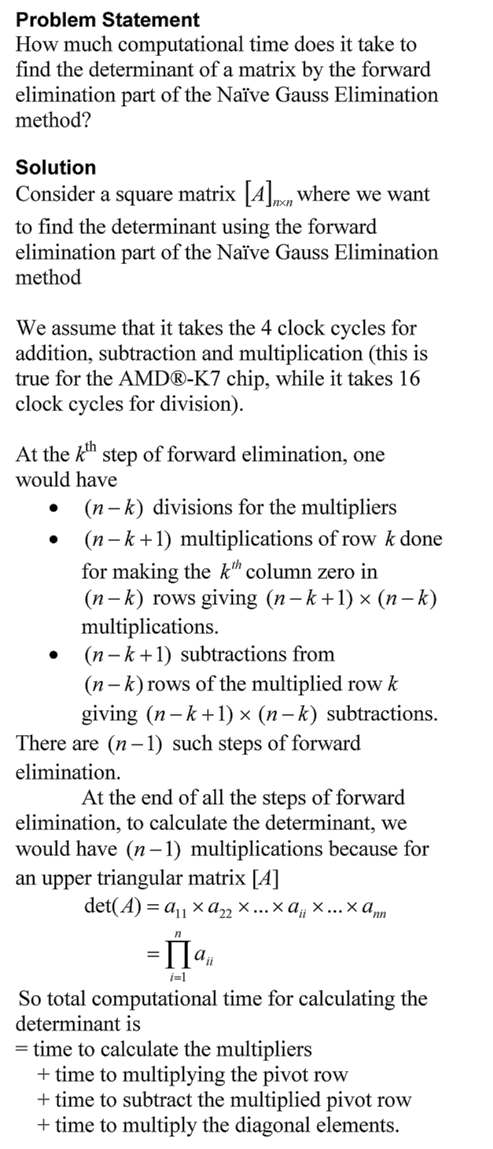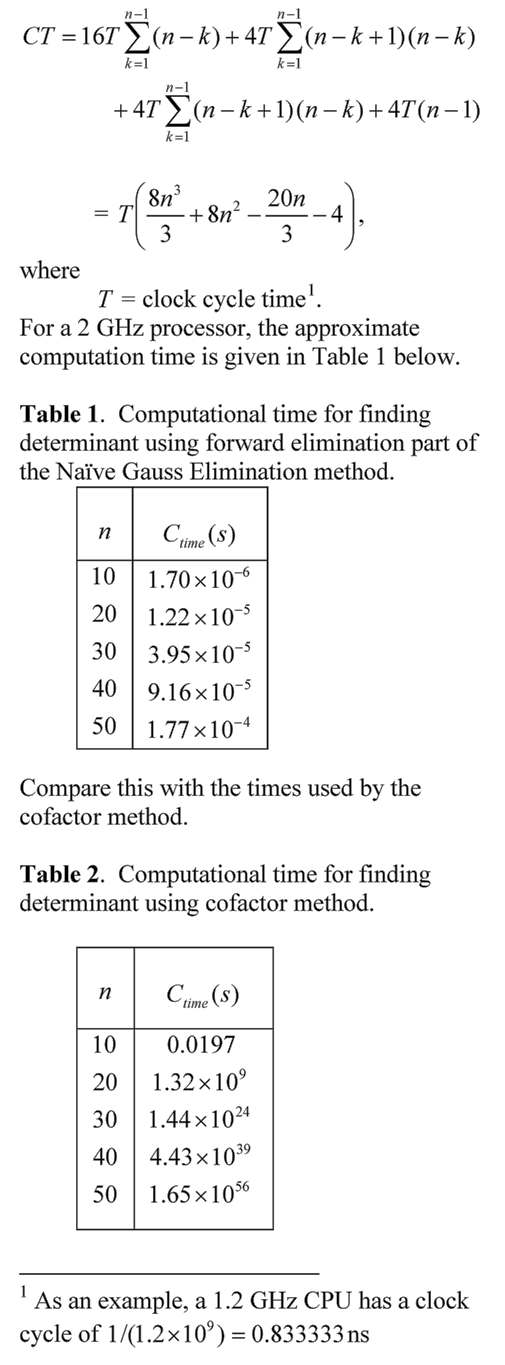## Matrix Algebra: Gaussian Elimination Method

Many university STEM major programs have reduced the credit hours for a course in Matrix Algebra or have simply dropped the course from their curriculum.   The content of Matrix Algebra in many cases is taught just in time where needed.  This approach can leave a student with many conceptual holes in the required knowledge of matrix algebra. In this series of blogs, we bring to you ten topics that are of immediate and intermediate interest for Matrix Algebra. Here is the sixth topic where we talk about solving a set of simultaneous linear equations using Gaussian elimination method – both Naive and partial pivoting methods are discussed. How to find determinants by using the forward elimination step of Gaussian elimination is also discussed.  Get all the resources in form of textbook content, lecture videos, multiple choice test, problem set, and PowerPoint presentation. Gaussian Elimination Method
This post is brought to you by
• Holistic Numerical Methods Open Course Ware:
• the textbooks on
• the Massive Open Online Course (MOOCs) available at

## Computational Time for Forward Elimination Steps of Naive Gaussian Elimination on a Square Matrix

Problem Statement

How much computational time does it take to conduct the forward elimination part of the Naïve Gauss Elimination method on a square matrix?This post is brought to you by

• Holistic Numerical Methods Open Course Ware:
• the textbooks on
• the Massive Open Online Course (MOOCs) available at

## Computational Time to Find Determinant Using Gaussian Elimination

The time it would take to find the determinant of a matrix using the Gaussian Elimination is many-many orders less than when the cofactor method is used.  In this blog, we derive the formula for a typical amount of computational time it would take to find the determinant of a nxn matrix using the forward elimination part of the Naive Gauss Elimination method.  The time is compared with that using the cofactor method.This post is brought to you by Holistic Numerical Methods: Numerical Methods for the STEM undergraduate at http://nm.mathforcollege.com, the textbook on Numerical Methods with Applications available from the lulu storefront, the textbook on Introduction to Programming Concepts Using MATLAB, and the YouTube video lectures available at http://nm.mathforcollege.com/videos.  Subscribe to the blog via a reader or email to stay updated with this blog. Let the information follow you.